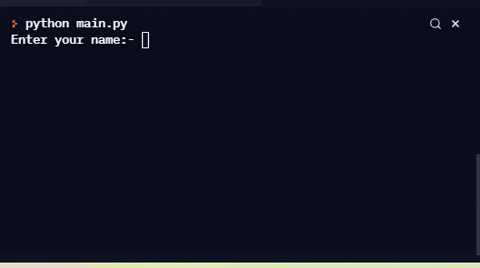## DEV Community is a community of 700,827 amazing developers

We're a place where coders share, stay up-to-date and grow their careers.

# Guess the Number Game in PythonDrTime

Today, you will be learning on creating a simple console guessing number game in python. It is pretty fun and easy to make. Make sure you try this too!

First of all, we make a file(has to have .py extension after the file name). Then, our code wants to be polite with the user. So, it wants the user to put his/her name! So, we can do a simple code below

``````user_name = input("Enter your name:- ")
user_name.strip()
``````

This is quite simple. We just take the user name as input and strip it.

Then, we want an option method. So, for example the game will say "Hello user_name, Do you want to play the game?" and there will be options for yes and no.

``````print(f"Hello {user_name}!")
print("Would you like to play Guess the number game?")
print("1) Yes")
print("2) No")
option = int(input("Select your option:- "))
``````

You should understand what I have written above.

Now, after all the formalities we jump into the main part. So, the game or code will get a random number from upper bound and lower bound(which we will come later). So we import random.

``````import random
``````

We also want tries or chances system for guessing the number.

``````tries = 0
``````

Our job now is to do the options work. So, if the user inputs option no. 1 i.e. yes then the following code will do the job for you.

``````if option == 1:
lower = int(input("Enter the lower bound:- "))
upper = int(input("Enter the higher bound:- "))
number = random.randint(lower, upper)
print(f"I am thinking a number in between {lower} and {upper}.")
print("You have to guess the correct number in 3 tries")
guess = int(input("Guess the number:- "))
tries+=1
if guess > number:
print("Guess lower...")
if guess < number:
print("Guess higher...")
while guess != number and tries < 3:
guess = int(input("Try again: "))
tries += 1
if guess > number:
print("Guess lower...")
if guess < number:
print("Guess higher...")
if guess == number:
print("You won!")
print(f"Number of tries:- {tries}")
else:
print("You lost!")
print(f"The number was {number}")
``````

Let me explain this. So, firstly we take the upper and lower bound from the user. Then we find a random number between them using random module. Then, we take the input of the guess given by the user. We give hints if the user guessed the number higher than the number that is to be guessed or vice-versa. And if the guess of the user is equal to he random number by the code before 3 chances, the player wins. And if not, then they have to reload the game and it will start from the start.

We already did our main code. Now, we do the following stuffs.

``````elif option == 2:
print("Thank you!")
else:
print("Invalid Option")
``````

If user inputs the second option which is no, then the game ends. And, the user gives anything other than 1 or 2, the code will say that it was an invalid option.
Here is the game example below:-The whole code is available at:
Github: https://github.com/CodeFictions/guessing-the-number-game